# Strain Gage

Also found in: Dictionary, Thesaurus, Medical, Acronyms, Wikipedia.
Related to Strain Gage: LVDT

## strain gage

[′strān ‚gāj]
(engineering)
A device which uses the change of electrical resistance of a wire under strain to measure pressure.
McGraw-Hill Dictionary of Scientific & Technical Terms, 6E, Copyright © 2003 by The McGraw-Hill Companies, Inc.
The following article is from The Great Soviet Encyclopedia (1979). It might be outdated or ideologically biased.

## Strain Gage

(also extensometer), a measuring transducer that converts the deformation (strain) of a solid induced by mechanical stress into a signal, usually an electrical signal; the signal is subsequently transmitted, converted, and recorded. Resistance strain gages, the most common type, use resistors whose electrical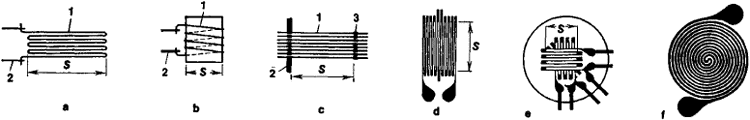Figure 1. Strain gage grids: (a) looped wire, (b) coiled wire, (c) cross-connected wire, (d) foil grid for measuring one deformation component, (e) foil grid for measuring three deformation components (f) foil arid for measuring circular deformations; (1) wire, (2) grid leads, (3) cross connectors; (S) gage base

resistivity changes as a result of deformations (expansion or compression). The resistors are constructed in the form of wire or foil grids (Figure 1, page 172) made of constantan, Nichrome, or various alloys of Ni, Mo, or Pt or in the form of thin sheets of a semiconductor material, such as silicon.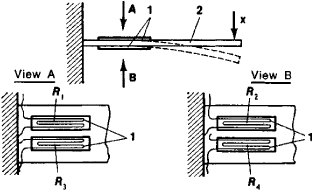Figure 2. Diagram of resistance strain gage: (1) grid, (2) elastic element; (R1), (R2), (R3), and (R4) strain-sensitive resistors; (x) measured parameter

Resistors may be rigidly bonded (glued or welded) to the elastic element of the strain gage (Figure 2) or attached directly to the object being studied. The elastic element senses changes in a given parameter x (pressure, deformation of a machine part, or acceleration) and converts the changes into a deformation ∊(x) of the grid or sheet. This deformation changes the resistance of the resistor by the amount ∆R(∊) = ±kR0, where R0 is the initial resistance of the resistor and k is the coefficient of strain sensitivity (k ≤ 2–2.5 for a wire strain gage, and k ~ 200 for semiconductor strain gages). Resistance strain gages usually operate within the elastic limit, where ∊ ≤ 10-3.

The magnitude of ∆R depends not only on ε but also on the temperature of the elastic element: ∆R(θ) = α∆θ R0, where ∆θ is the temperature change of the elastic element and a is the temperature coefficient of relative change in the resistance of the resistor. For wire and foil resistors, ot ranges between 2 × 10-3/°K and 7 × 10-3/°K.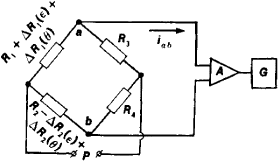Figure 3. Diagram of two strain-sensitive resistors in a bridge circuit: R1 + ∆R1 (∊) + ∆R1 (θ) and R2 — ∆R2(∊) + ∆R2(θ) are the resistances of the strain-sensitive resistors, where ∆R(∊) and ∆R(θ) are the changes in resistance of the strain-sensitive resistors, dependent on the change in deformation and the change in temperature θ, respectively. R3 and R4 are resistances of conventional resistors, and iah is the current in the bridge diagonal. (P) is the power source, (A) is the amplifier, and (G) is the recording device.

Temperature compensation or automatic introduction of temperature corrections is needed to decrease measurement errors. The most commonly used temperature compensation circuits use bridge circuits. Figure 3 shows an example of two identical strain-sensitive resistors incorporated in a bridge circuit and designed to sense the deformation of an elastic element. In this instance, ∆R1(∊) and ∆R2(∊) have opposite signs, whereas ∆R1(θ) and ∆R2(θ) have the same sign. When ∆R/R << 1, the current along the bridge diagonal, which constitutes the output signal of the strain gage, is given by the expression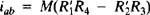, where M is a proportionality coefficient and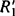and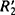, the resistances of the strain-sensitive resistors, are equal to R1 + ∆ + R1(∊), + ∆R1(θ) and R2 – ∆R2(∊) + ∆R2(θ), respectively. Compared with bridge circuits having a single resistor, those with two resistors double the sensitivity of the strain gage, and those with four resistors quadruple the sensitivity, thus ensuring complete temperature compensation.

### REFERENCES

Turichin, A. M. Eleklricheskie izmereniia neelektricheskikh velichin, 4th ed. Moscow-Leningrad, 1966.
Glagovskii, B. A., and I. D. Piven. Elektrotenzometry soprotivleniia, 2nd ed. Leningrad, 1972.

A. V. KOCHEROV

## Strain gage

A device which measures mechanical deformation (strain). Normally it is attached to a structural element, and uses the change of electrical resistance of a wire or semiconductor under tension. Capacity, inductance, and reluctance are also used.

The strain gage converts a small mechanical motion to an electrical signal by virtue of the fact that when a metal (wire or foil) or semiconductor is stretched, its resistance is increased. The change in resistance is a measure of the mechanical motion. In addition to their use in strain measurement, these gages are used in sensors for measuring the load on a mechanical member, forces due to acceleration on a mass, or stress on a diaphragm or bellows.

McGraw-Hill Concise Encyclopedia of Engineering. © 2002 by The McGraw-Hill Companies, Inc.
References in periodicals archive ?
As described by Alan Szary, vice president, engineering and business development at the company, "Precision Filters' strain conditioning cards employ our proprietary BCC technology that excites the strain gage using constant current rather than constant voltage excitation, eliminating measurement inaccuracies related to lead wire resistance.
Hole-Drilling Strain Gage Method of Measuring Residual Stresses, Experimental Mechanics, Vol 6, No.
Such verification could consist of applying a strain gage rosette, identical to the rosette used in the test, to a stress-free specimen of the same nominal composition, and then drilling a hole.
When a strain gage replaces one or more of the resistors in the bridge, and as the strain gage undergoes dimensional changes because it is bonded to a test specimen, it unbalances the bridge and produces an output voltage proportional to the strain.
Strain measurements on wood materials using directly applied electrical strain gages (SG) have been successfully carried out at various research facilities in the past.
The sensors are made by fusing silicon strain gages at high temperature with inorganic glass to the load-measuring member.
The least amount of change to the construction and the mechanical properties of the mops can be handled by the use of strain gages, such as those manufactured by HBM, Marlboro, MA.
Pressure is applied directly to the sensing diaphragm where bonded loll strain gages measure the induced strain.
That is, an NVH system will include such sensors as accelerometers, strain gages, force sensors, etc., and multiples of each.
The MGCplus acquires data from a variety of sensors placed on the rocket, including strain gages, thermocouples, load cells and LVTDs, and presents it to engineers in native or engineering units.

Site: Follow: Share:
Open / Close